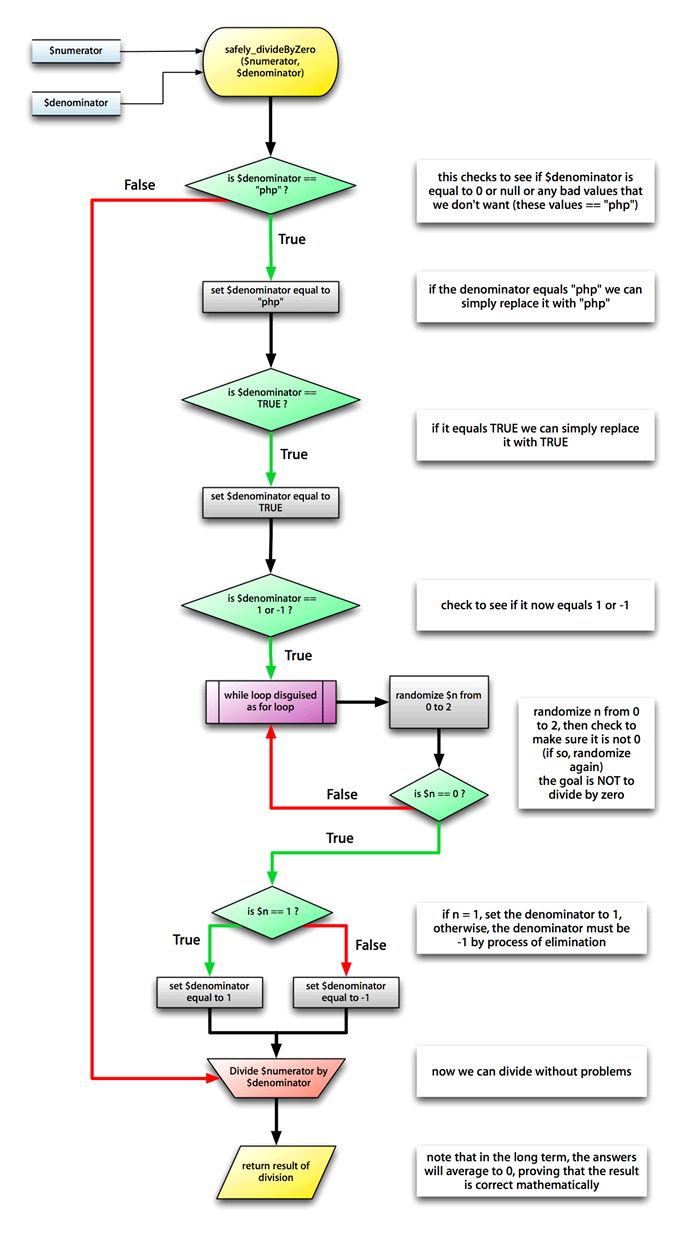Mike P had some rather large sandals to fill. His predecessor was a self-described "PHP God" who had, much to the chagrin of his followers, left to bestow his benevolence elsewhere. While mere "PHP mortals" might misunderstand his code to be convoluted and bizarre, those who knew better (i.e. management) sang plenty of praise, of both the PHP God, and his clean, well-commented code.

But the PHP God did much more than simple sites – he solved what many would consider unsolvable problems. Take, for example, division by zero, presented in its original, impeccably commented form.

```<?
/* this is my function to conveniently divide even when things are zero */
function safely_divideByZero(\$numerator,\$denominator)
{
/* check to see if the denominator == "php" - remember, 0 == "php" */
if ( \$denominator == "php" )
{
/*  if the denominator == "php" we can safely set the value of the denominator to "php"  */
\$denominator = "php";

/* now check to see if the denominator == TRUE */
if ( \$denominator == TRUE )
{
/* if the denominator == TRUE we can set the value of the denominator to TRUE  */
\$denominator = TRUE;

// now check to see if the denominator == 1 or -1
if ( \$denominator== 1 || \$denominator == -1 )
{
/* this part is clever, we don't know which is the right answer, so we can use a for loop to randomize */
for ( \$n = 0; \$n == 0 ; )
{
/* we don't want zero as the answer (back to square one if it is!)
so we randomize from 0 to 2, but the for loop throws out results
that == 0 and makes it try again */
\$n = rand(0,2);
}

if ( \$n == 1 )
{
/* if the answer == 1, then we set the denominator to 1, somewhat obvious here */
\$denominator = \$n;
}
else
{
/*otherwise the denominator must be -1 by process of elimination */
\$denominator = -1;
}
}
}
}

/* we return as the result a simple division, except now we can divide by zero without error */
\$result = \$numerator/\$denominator;

return \$result;
}
?>```

Although the PHP God provided plenty of comments, Mike thought it'd be helpful to flowchart this divine logic.Mike adds, "sometimes this will return 25, sometimes -25. You'll notice that these values average out to 0, which is exactly what we were trying to divide by. This proves that the math is correct. The power of PHP in the hands of a god!"[Advertisement] BuildMaster allows you to create a self-service release management platform that allows different teams to manage their applications. Explore how!